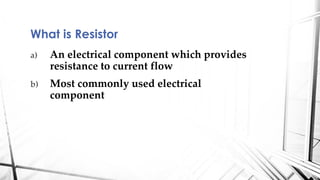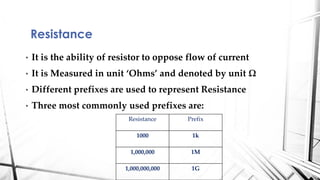Ce diaporama a bien été signalé.
Le téléchargement de votre SlideShare est en cours. ×

# Resistors - Electrical Engineering

Publicité
Publicité
Publicité
Publicité
Publicité
Publicité
Publicité
Publicité
Publicité
Publicité
PublicitéChargement dans…3
×

## Consultez-les par la suite

1 sur 24 Publicité

# Resistors - Electrical Engineering

An excellent guide on resistors which provides knowledge on Types, Color coding, Carbon film, Metal film, prefixes, symbols, wire wound, integrated circuit resistors, Color coding, potentiometer, negative temperature coefficient, positive temperature coefficient, series, parallel, series-parallel configurations and the practical method to measure the value of resistance. The concept of Ohm Law is also introduced. Formulas for solutions are also provided. The coding method is explained with the help of chart and all 4-band, 5-band, 6-band types are discussed.

An excellent guide on resistors which provides knowledge on Types, Color coding, Carbon film, Metal film, prefixes, symbols, wire wound, integrated circuit resistors, Color coding, potentiometer, negative temperature coefficient, positive temperature coefficient, series, parallel, series-parallel configurations and the practical method to measure the value of resistance. The concept of Ohm Law is also introduced. Formulas for solutions are also provided. The coding method is explained with the help of chart and all 4-band, 5-band, 6-band types are discussed.

Publicité
Publicité

### Resistors - Electrical Engineering

1. 1. An absolute guide from ABC to XYZ. Resistor
2. 2. a) An electrical component which provides resistance to current flow b) Most commonly used electrical component What is Resistor
3. 3. Resistance • It is the ability of resistor to oppose flow of current • It is Measured in unit ‘Ohms’ and denoted by unit Ω • Different prefixes are used to represent Resistance • Three most commonly used prefixes are: Resistance Prefix 1000 1k 1,000,000 1M 1,000,000,000 1G
4. 4. Symbols 1. Internationally a zig-zag model is used to represent the resistors. 2. Whereas in UK most people use the symbol on left.
5. 5. Types of Fixed Resistors ( Design Models) Carbon film Metal film Metal Oxide Wire wound resistors
6. 6. Types of Resistors Potentiometer It provides variable resistance. Phototransistor Tapped Resistor
7. 7. • These are inexpensive and are very easily available resistors. • These resistors are available in Ranges 1Ω to 100 MΩ • The power ratings are in between 0.125-2W. Cons • There is a large tolerance in rating and mostly 5-10% difference is expected. • Such resistors are recommended for small applications like experiments in labs. But are not recommended for large applications. Carbon Film Resistors
8. 8. • They re expensive compared to Carbon Film resistors • Are highly recommended for projects Metal Film Resistors
9. 9. • They have special ability to dissipate large quantities of heat. • These are recommended when there is large variation in temperatures. • Such resistors contain porcelain which is covered by metal (usually steel or some kind of mixed steel alloy). • This steel alloy is then again coated with porcelain • Remember that porcelain has property to absorb heat through conductors. Wire wound resistors
10. 10. • Such resistors are enclosed in IC’s • They are expensive as compared to single resistor but are quite easy to manage. • Can be used where you require 4 or more resistors and less space is available Integrated Resistors
11. 11. • They provide variable resistance. • Are required when you need variable values, for example you may need 7k ohm. • Have large values of applications in motor speed control, fan speed control, radio frequecnies. Variable Resistor (Potentiometer) Symbol Practical Look Working
12. 12. • Its resistance depends on the temperature • If temperature changes the value of resistance changes. Thermistor How it looks Symbol
13. 13. • NTC stands for negative temperature coefficient. • The resistance of such resistors decreases with increase in temperature. • Such resistors are installed in parallel NTC Thermistor (Continue) Resistancealongy-axis Temperature along x-axis
14. 14. • PTC stands for positive temperature coefficient • The resistor of such components increases with temperature • They are mostly loaded in parallel PTC Thermistor Continue Resistancealongy-axis Temperature along x-axis
15. 15. • Used to identify the values of resistors. • A color code chart is used for reference. Resistor Color coding
16. 16. • Resistors in circuits can be arranged in three configurations 1.Series 2.Parallel 3.Series-Parallel Resistor configurations
17. 17. • Two or more components are in series if the head of one connects with tail of another. • Image below displays three resistors that join in series to each other. Series Configuration
18. 18. • In Numerical analysis you are mostly required to solve all resistors to simple single resistor. • To solve resistors in series simply add them: Series Configuration (continue)
19. 19. • Two resistors are in parallel when their heads and tails are joined together. Parallel Configuration
20. 20. • To solve parallel resistors the formula used is: Parallel Resistors (Continue)
21. 21. • Very often complex circuit contain series-parallel configurations. • However you can easily solve them if you learn the basic concept. Series-Parallel
22. 22. • You need a ohmmeter to measure it practically. • Connect Ohmmeter in parallel to the resistor and it will display the value. How to measure Resistance???
23. 23. • In numerical problems you can find the resistance provided voltage and current is given. • Use Ohm Law for this purpose. R = V/I Resistors & Ohm Law
24. 24. • Guzel Sansilaniz is an Electrical Educationist, Design Engineer (Siemens) , Part time teacher (working in a school (voluntarily) too), student (wow). • I am learning and I will share all I learn, • Join my Facebook group or Slide share account for future updates. Links are all given. Or simply copy this url in browser: https://goo.gl/3CUHbH About Me